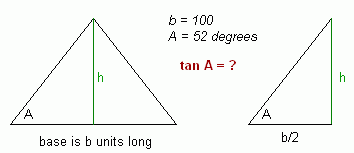SEARCH HOMEMath Central Quandaries & QueriesSubject: finding the height of a triangle Name: Brendan Who are you: Student I need to find the height of an isosceles triangle whose angles are 52, 52 and 76 degrees. The base is 100, and the two equal sides are unknown. How would I go about this?Hi Brendan.

The easiest way is to draw a line from the corner with the large angle to the opposite side. Because this is an isosceles triangle, this line divides the triangle into two congruent right triangles. As well, this line you've drawn is the height of the original triangle.

Now you have a right triangle and you know the measure of the angle opposite the height and you know the length of the side (half the base b).

You can use a trigonometric tangent function to relate the known values to the unknown values.Does this help?
Stephen La Rocque.Math Central is supported by the University of Regina and The Pacific Institute for the Mathematical Sciences.